# Area of Square

Go back to  'Area'

 1 Introduction to Square 2 What is a Square? 3 Definition of Area of a Square 4 Area of a Square Formula 5 Sample Problems on Area of a Square 6 Area of a Square Calculator 7 Important Notes on Area of a Square 8 Practice Questions on Area of a Square 9 Common Mistakes 10 Challenging Questions on Area of a Square 11 Maths Olympiad Sample Papers 12 Frequently Asked Questions (FAQs)

We at Cuemath believe that Math is a life skill. Our Math Experts focus on the “Why” behind the “What.” Students can explore from a huge range of interactive worksheets, visuals, simulations, practice tests, and more to understand a concept in depth.

Book a FREE trial class today! and experience Cuemath’s LIVE Online Class with your child.

## Introduction to Square

A square is a closed two-dimensional shape with four equal sides and four equal angles.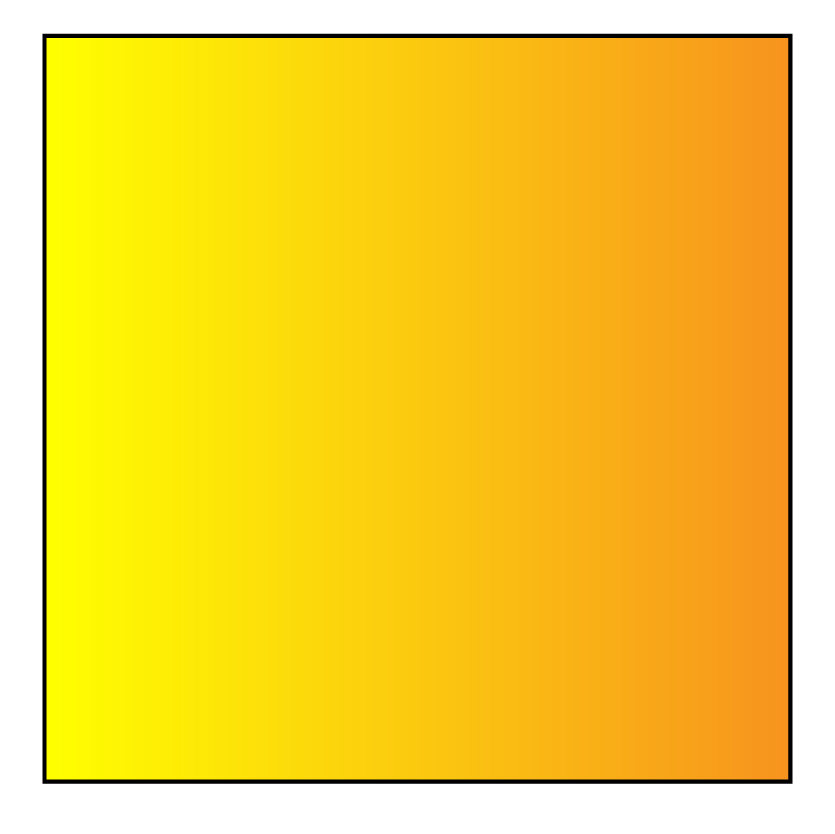Squares are all around us.

Here are some commonly seen items which are square.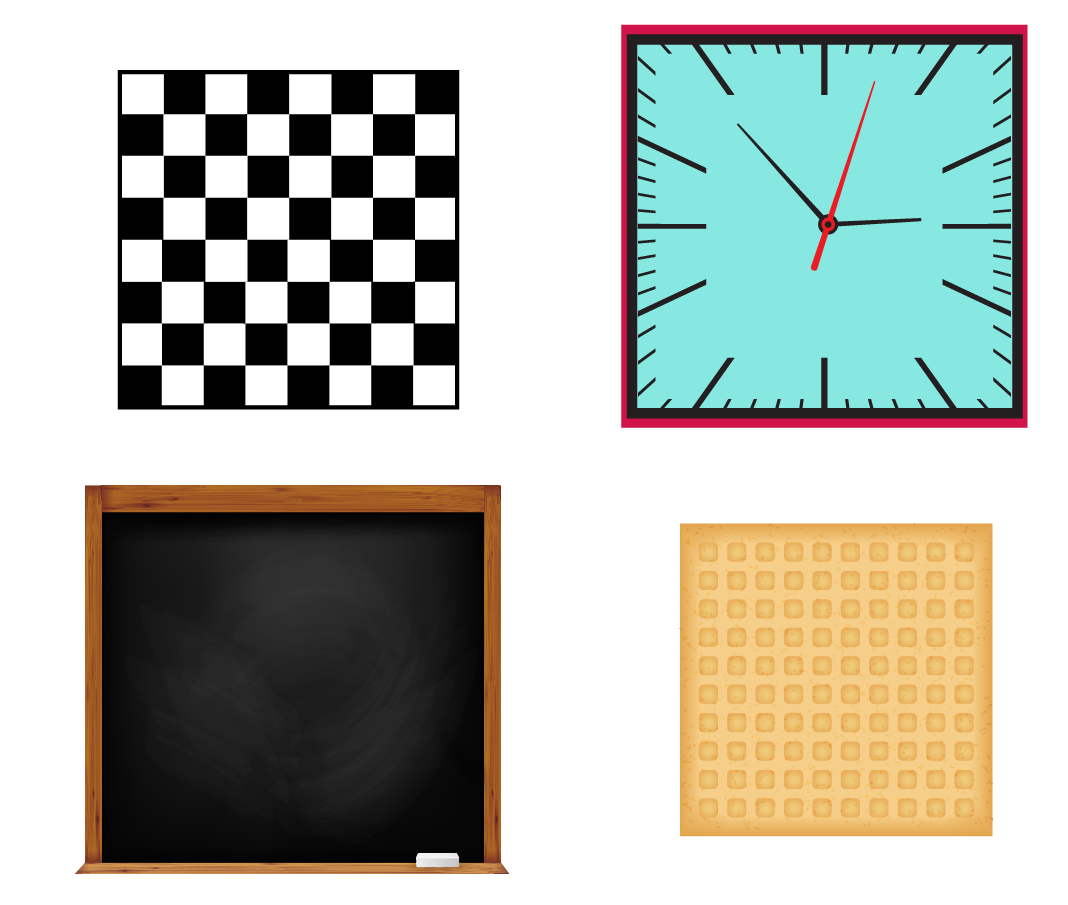## What is a Square?

It is a quadrilateral in which:

• the opposite sides are parallel.
• all four sides are equal.
• all angles measure 90$$^\circ$$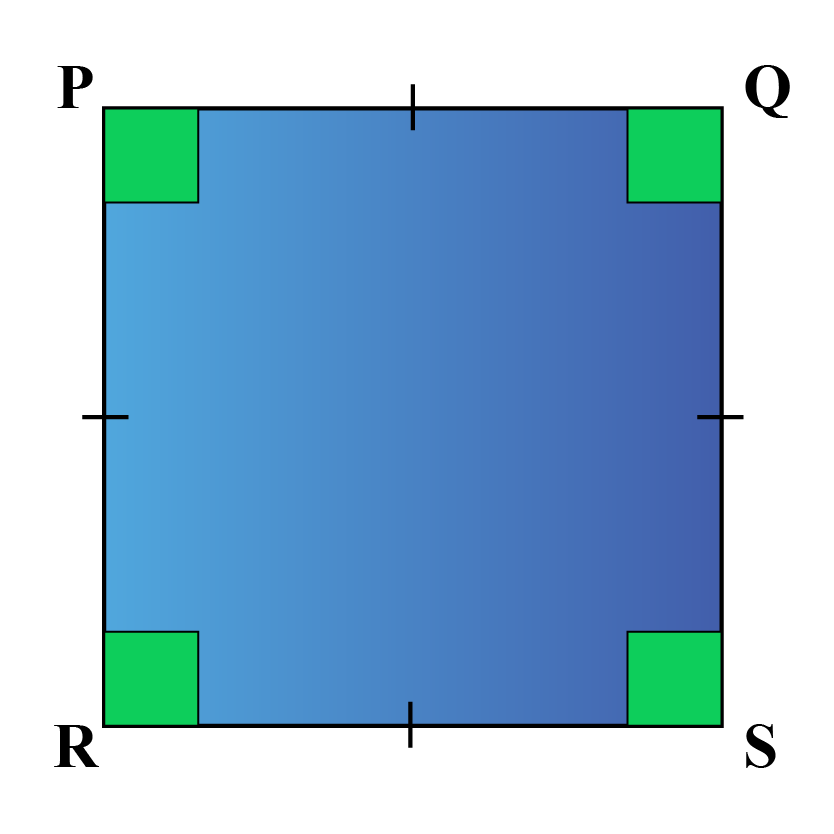Drag the vertex in the simulation below.

You can observe that all four sides in a square are equal and all the angles are 90$$^\circ$$

## Definition of Area of a Square

The area of a square is the space occupied by the square.

Look at the green square shown below.

It has occupied 25 square units.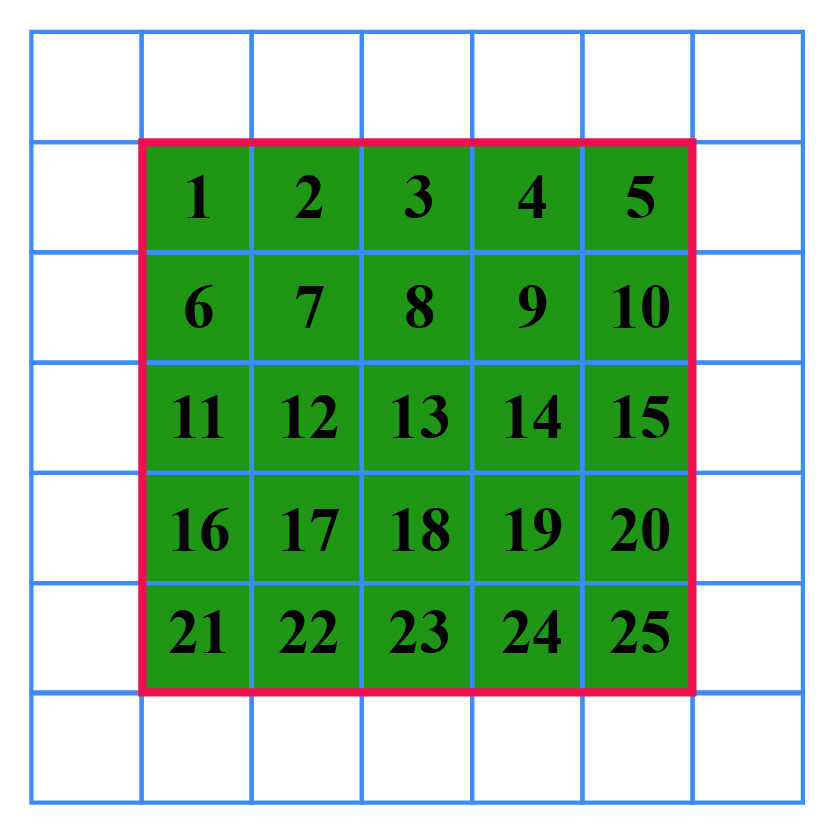Therefore, the area of the square is 25 square units.

From the figure, we can observe that the length of each side is 5 units.

Therefore, the area of the square is the product of its sides.

Area of square = side $$\times$$ side = 25 square units

## Area of a Square Formula

The formula for the area of a square with side '$$s$$' is: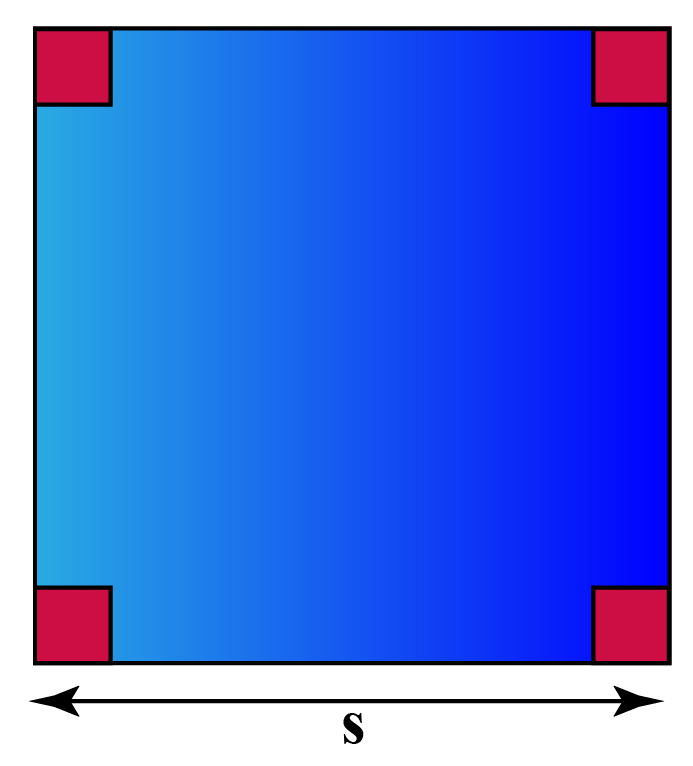\begin{align} \text {Area of a Square} = s \!\times\! s \text{ units}^2 \end{align}

To understand this better, let us look at this solved example which uses the area of a square formula.

Find the area of a square of side 7 $$cm$$.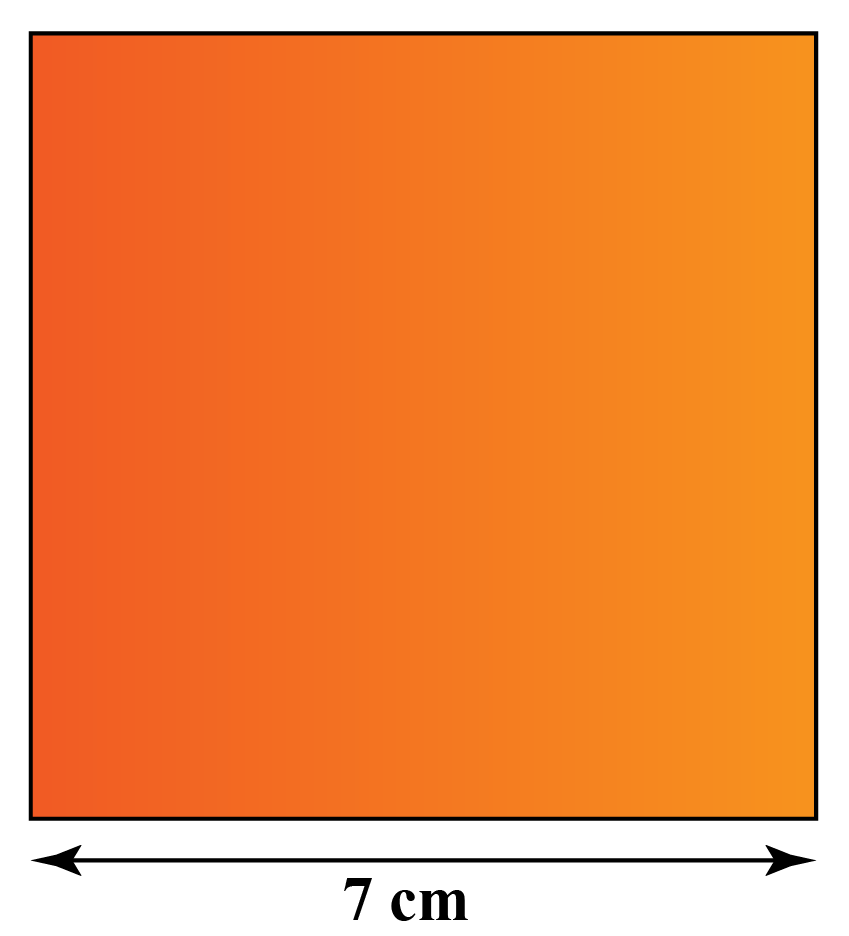Area of a square of side 7 $$\text{cm}$$ is

\begin{align} 7 \text{ cm} \times 7 \text{ cm}= 49 \;\text{cm}^2 \end{align}

When the diagonal $$d$$ of the square is known, the formula for finding the area of the square is: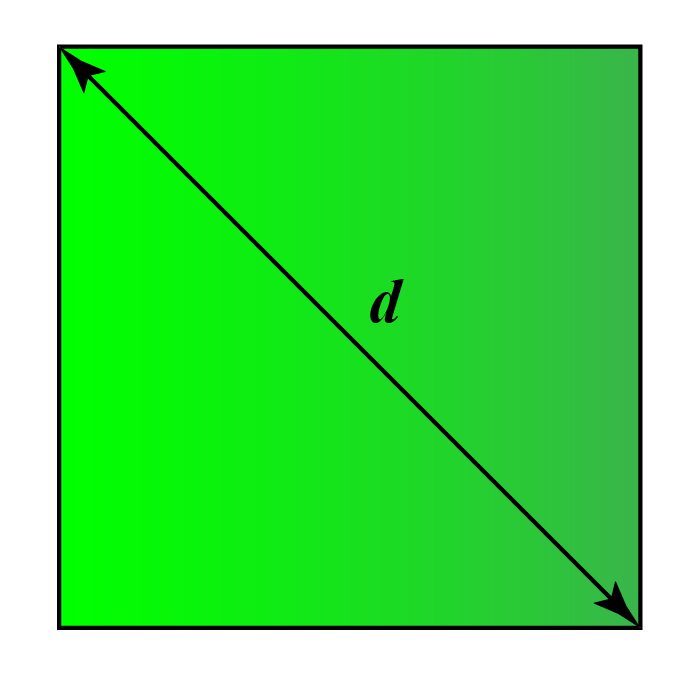\begin{align} \text {Area of a Square} = \frac{d^2}{2} \end{align}

You will understand these concepts better from the following solved examples for the area of a square formula.

Help your child score higher with Cuemath’s proprietary FREE Diagnostic Test. Get access to detailed reports, customised learning plans and a FREE counselling session. Attempt the test now.

## Area of a Square Sample Problems

 Example 1

What is the area of a square swimming pool of side 8 $$m$$?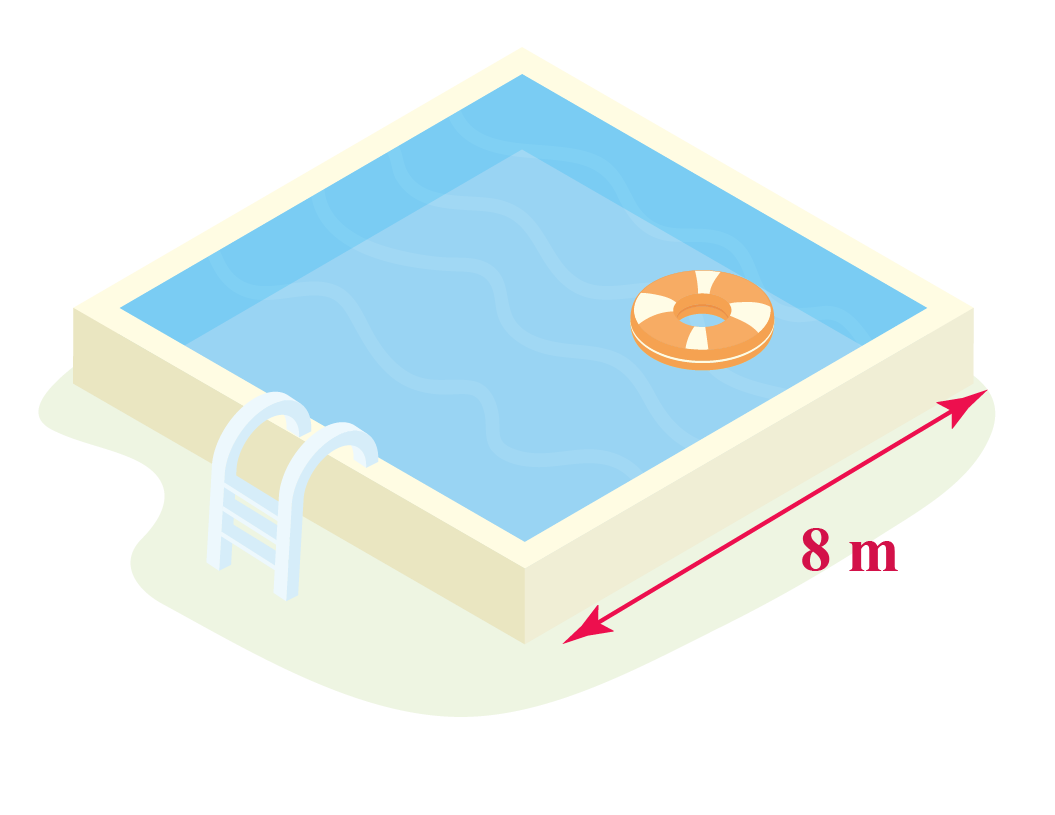Solution:

We know that the area of a square of side $$s$$ is $$s^2$$

Therefore, the area of the swimming pool is

\begin{align} 8 \times 8 = 64 \: m^2\end{align}

 $$\therefore$$ Area of the swimming pool = 64 $$m^2$$
 Example 2

The area of a square carrom board is 3600 $$cm^2$$. What is the length of its sides?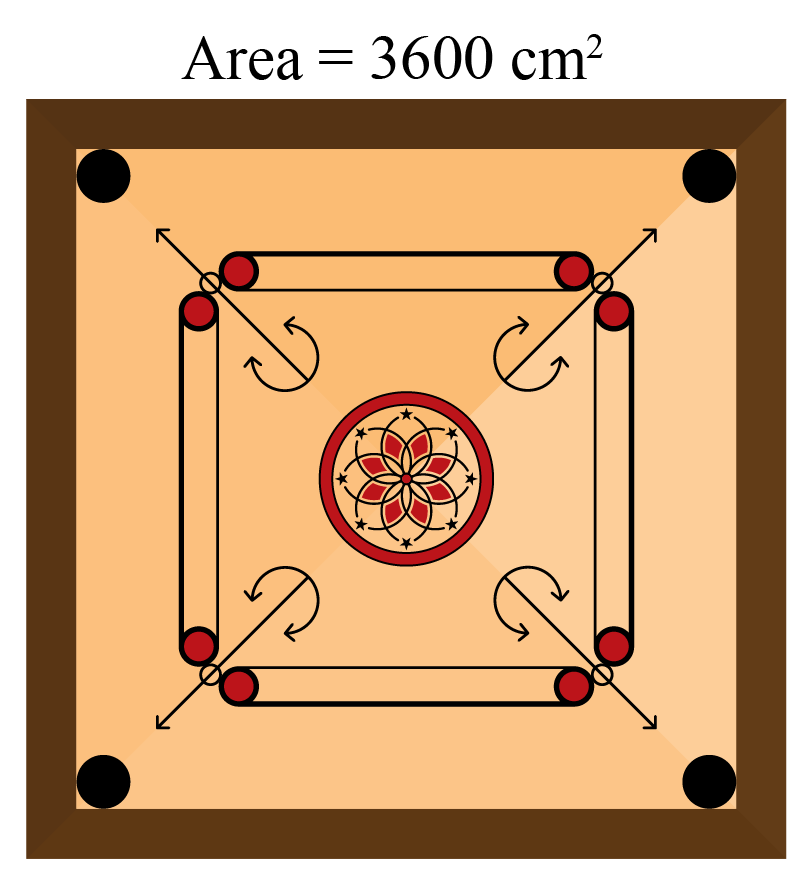Solution:

Area of a square of side $$s =s^2$$

Area of the square carrom board = 3600 $$cm^2$$

We know that 60 $$\times$$ 60 gives 3600

 $$\therefore$$ Side of the carrom board = 60 $$cm$$
 Example 3

Find the area of the floor of a square room which is made up of 100 tiles of side 5 $$cm$$.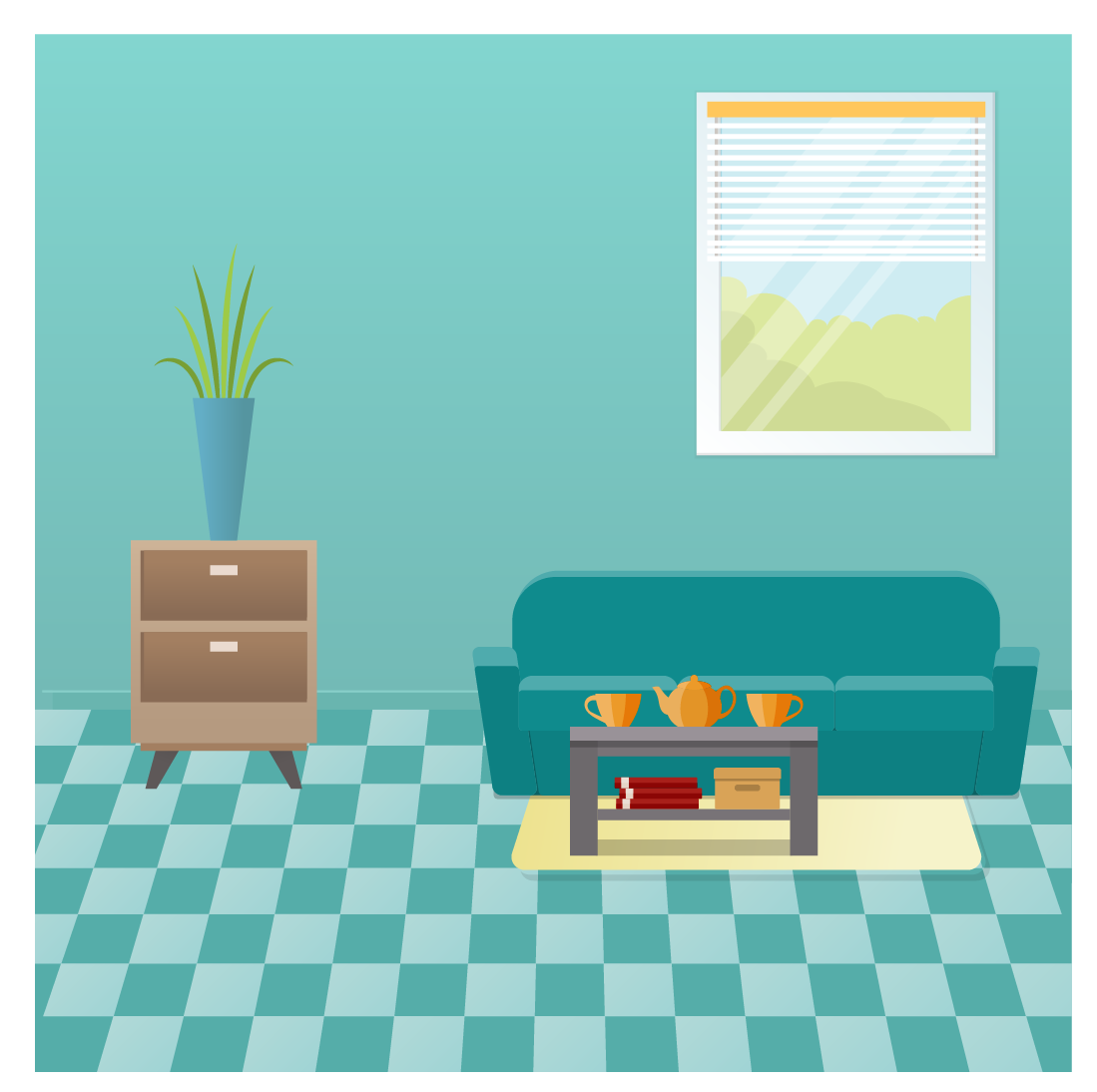Solution:

Area of one tile

\begin{align} &= 5 \text{ cm} \times 5 \text{ cm} \\ &= 25 \text{ cm}^2\end{align}

We know that there are 100 tiles on the floor of the room.

Thus, the area occupied by 100 tiles is the floor area

\begin{align} &= 100 \times 25 \text{ cm} \\ &= 2500 \text{ cm}^2 \end{align}

 $$\therefore$$ Area of the floor = 2500 $$cm^2$$
 Example 4

Find the area of a square carpet whose diagonal is 4 feet.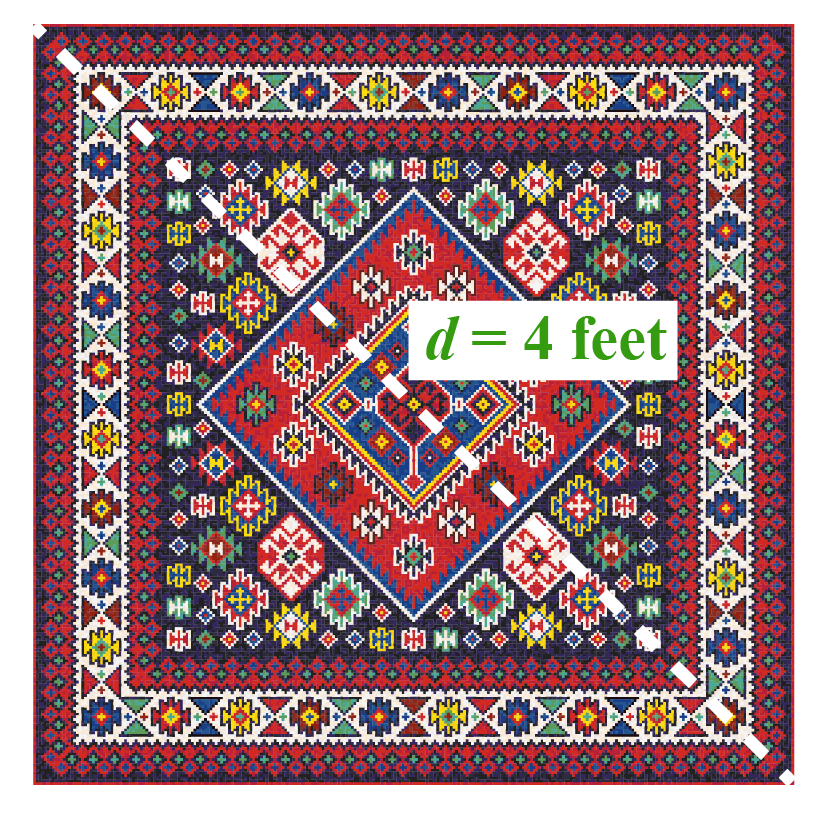Solution:

The area of a square when its diagonal is given is \begin{align} \frac{d^2}{2} \end{align}

Given, diagonal d = 4 ft

Area of the carpet

\begin{align} &= \frac{4 \times 4}{2} \\\\ &= \frac{16}{2} \\\\ &= 8\: \text{square feet} \end{align}

 $$\therefore$$ Area of the carpet = 8 square feet
 Example 5

A square wall of side 100 $$m$$ needs to be painted.

What will be the cost of painting, if the painter charges Rs. 2 to paint an area of 1 $$m^2$$Solution:

Area of the square wall

\begin{align} &= 100 \times 100 \\ &= 10,000 \: m^2 \end{align}

The cost of painting 1 $$m^2$$ is Rs. 2

Thus, the cost to paint an area of 10,000 $$m^2$$

$$10,000 \times 2 = 20,000$$

 $$\therefore$$ Cost of painting =  Rs. 20,000

## Area of a Square Calculator

Use the area of a square calculator to find the area of any given square.

Enter the value in the space provided and click on Go to view the answer.

CLUEless in Math? Check out how CUEMATH Teachers will explain Area of Square to your kid using interactive simulations & worksheets so they never have to memorise anything in Math again!

Explore Cuemath Live, Interactive & Personalised Online Classes to make your kid a Math Expert. Book a FREE trial class today!

## Practice Questions

Here are a few problems related to the area of a square formula.

## Common Mistakes

• A common mistake that we tend to do while calculating the area of a square is doubling the number.

This is incorrect!

Always remember that the area of a square is side $$\times$$ side and not 2 $$\times$$ side.
• Units have to be mentioned when we represent the area.

The side of a square is one-dimensional and the area of a square is two-dimensional.

Hence, the area of a square is always represented as square units.

For example, a square of side 3 units will have an area of $$3 \times 3 = 9$$ square units.Challenging Questions
1. The diagonals of two squares are in the ratio 3 : 5

What is the ratio of their areas?
2. A wire of length 150 $$cm$$ is bent to form a square and then to a circle.

Which shape will have a more area?

IMO (International Maths Olympiad) is a competitive exam in Mathematics conducted annually for school students. It encourages children to develop their math solving skills from a competition perspective.

## 1. What is the formula for finding the area of a square?

The area of a square of side $$s$$ units:

$$s \times s = \text{ units}^2$$

The area of a square when the diagonal $$d$$ of the square is given:

\begin{align} \frac{d^2}{2} \end{align} square units

## 2. How do you calculate the area of a square?

Area of a square of side $$s$$ units is

$$s \times s = \text{ units}^2$$

## 3. Give an example to show how the area of a square is calculated.

The area of a square of side $$s$$ units is

$$s \times s$$

Example: Area of a square of side 5 cm

= $$5\text{ cm} \times 5\text{ cm} = 25$$ $$cm^2$$

More Important Topics
Numbers
Algebra
Geometry
Measurement
Money
Data
Trigonometry
Calculus